Raster

The next cell makes a matrix of values between 0.08 and 1.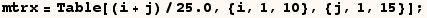In the next cell Raster makes an array of gray blocks with one block for each element of the matrix.  A value 0 in the matrix corrosponds to a black block in the graphic, and a value of 1 in the matrix corrosponds to a white block in the graphic.  Linear interpolation is used between 0.08 and 1. In this example we have a 10×15 matrix and the portion of the graphic covered with gray blocks goes from 0 to 15 in the horizontal direction, and from 0 to 10 in the vertical direction.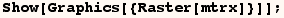In the next cell the Raster graphic is displayed over a red rectangle specified as  Rectangle[{-1,-1},{16,11}].  You can see that the gray blocks covers the range described above.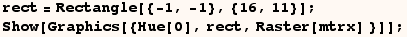In the next cell we give Raster the coordinates {{1,2},{14,9}} as a second argument.  Here the second argument of Raster indicates that the array of gray blocks should go from 1 to 14 in the horizontal direction and from 2 to 9 in the vertical direction.

I don't demonstrate it here but Scaled and Offset corrdinates can be used in the second argument given to Raster.  See another section for an explanation of Scaled, Offset.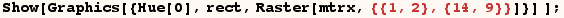Now consider the next cell where we make a matrix of numbers between  -1.8333 and 2.3333.  Raster treats negative matrix elements as 0 and treats matrix elements greater than 1 as 1.  As a result a lot of the array blocks are black or white.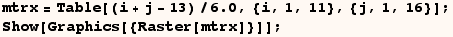In the next cell we use the same matrix with elements between -1.8333 and 2.3333, and we give Raster {-1.9, 2.4} as a third argument.  This says a value of  -1.9  in the matrix should make a black cell, a value of  2.4  in the matrix should make a white cell.  Linear interpolation is used for matrix elements between  -1.9  and  2.4.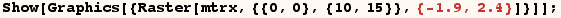In the next cell we give Raster {-3, 3} as a third argument.  This says a value of -3 in the matrix should make a black cell, a value of 3 in the matrix should make a white cell, and a linear interpolation should be used between -3 and 3.  Since all elements of the matrix are between -1.8333 and 2.3333, all blocks in the array are in the middle range of the gray scale.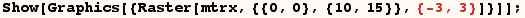In the next cell  (rstr1)  uses black if a matrix element is  -1.9,  and uses white if a matrix element is  2.4.  But then  (rstr2)  uses black if a matrix element is  2.4, and uses white if a matrix element is  -1.9.  As a result the shades in (rstr1) are the complement of the shades in (rstr2).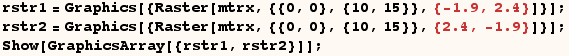Raster has options ColorFunction and ColorFunctionScaling which are explained in another section.

Created by Mathematica  (May 16, 2004)

Back to Ted’s Tricks index page